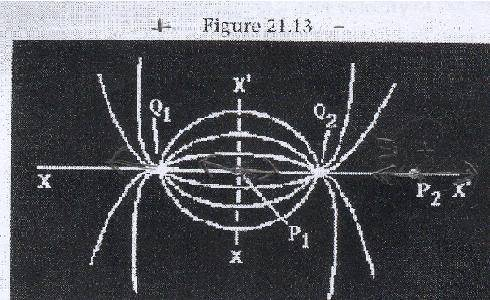# Electric Field lines problem

Hello Everyone!
Here is the link for the picture...From the figure, the electric Field lines arising from two charges Q1 and Q2 are shown. From this drawing we can see that
A) The electric field could be zero at P2
B) Both Q1 and Q2 have the same sign
C) |Q1|>|Q2|
D) the electric field could be zero at P1
E) none of these is true

Something is telling me that B is not true... why?
the lines go from one to the other, if they were from the same sign the lines would go apart from one another...now C... we can't tell because we don't know which one is proton and which is the electron. Choice E i don't think so..
So it must be either A or D, but i don't know which one and why...
can anyone help me here please?

Thanks!

I agree, B is not true. If the system contained a net charge- then there would be field lines that 'escape' from the 2 charges, whereas you can see that every field line is reabsorbed (Gauss's law).

can anyone give me a hint?

Assume one of them to be positive and the other, negative (since B is incorrect and none of them is zero charged). At P1 their fields add up, while at P2 they oppose each other. So, for some point lying in the region of P2, E will be zero.